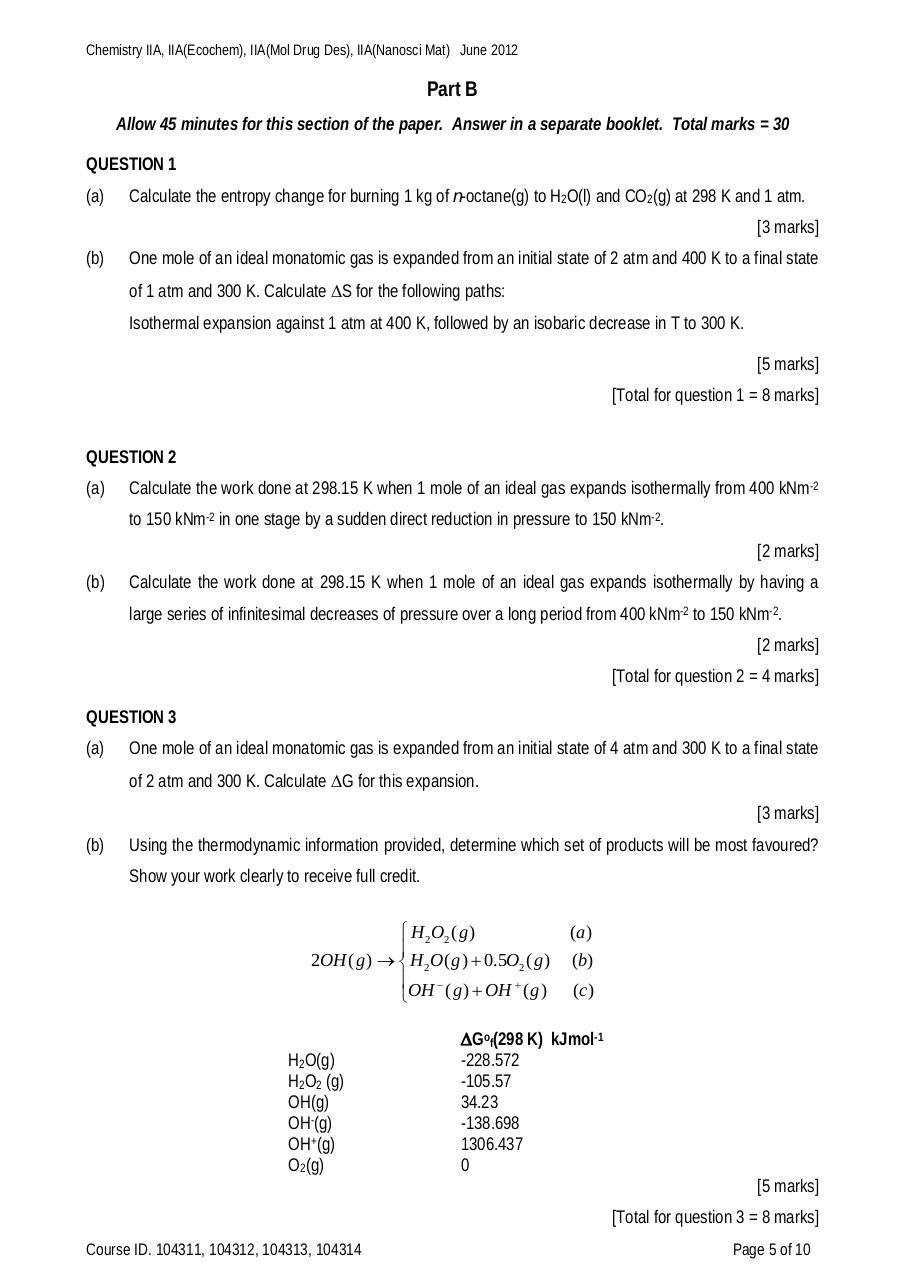# ChemIIA 2012 final.pdfPage 1...3 4 56717

#### Text preview

Chemistry IIA, IIA(Ecochem), IIA(Mol Drug Des), IIA(Nanosci Mat) June 2012

Part B
Allow 45 minutes for this section of the paper. Answer in a separate booklet. Total marks = 30
QUESTION 1
(a)

Calculate the entropy change for burning 1 kg of n-octane(g) to H2O(l) and CO2(g) at 298 K and 1 atm.
[3 marks]

(b)

One mole of an ideal monatomic gas is expanded from an initial state of 2 atm and 400 K to a final state
of 1 atm and 300 K. Calculate S for the following paths:
Isothermal expansion against 1 atm at 400 K, followed by an isobaric decrease in T to 300 K.
[5 marks]
[Total for question 1 = 8 marks]

QUESTION 2
(a)

Calculate the work done at 298.15 K when 1 mole of an ideal gas expands isothermally from 400 kNm-2
to 150 kNm-2 in one stage by a sudden direct reduction in pressure to 150 kNm-2.
[2 marks]

(b)

Calculate the work done at 298.15 K when 1 mole of an ideal gas expands isothermally by having a
large series of infinitesimal decreases of pressure over a long period from 400 kNm-2 to 150 kNm-2.
[2 marks]
[Total for question 2 = 4 marks]

QUESTION 3
(a)

One mole of an ideal monatomic gas is expanded from an initial state of 4 atm and 300 K to a final state
of 2 atm and 300 K. Calculate G for this expansion.
[3 marks]

(b)

Using the thermodynamic information provided, determine which set of products will be most favoured?

 H 2O2 ( g )

2OH ( g )   H 2O( g )  0.5O2 ( g )

OH ( g )  OH ( g )
H2O(g)
H2O2 (g)
OH(g)
OH-(g)
OH+(g)
O2(g)

(a)
(b)
(c )

Gof(298 K) kJmol-1
-228.572
-105.57
34.23
-138.698
1306.437
0
[5 marks]
[Total for question 3 = 8 marks]

Course ID. 104311, 104312, 104313, 104314

Page 5 of 10